# Atomic Spectroscopy Introduction To The Textbook Atomic Astrophysics

• Slides: 13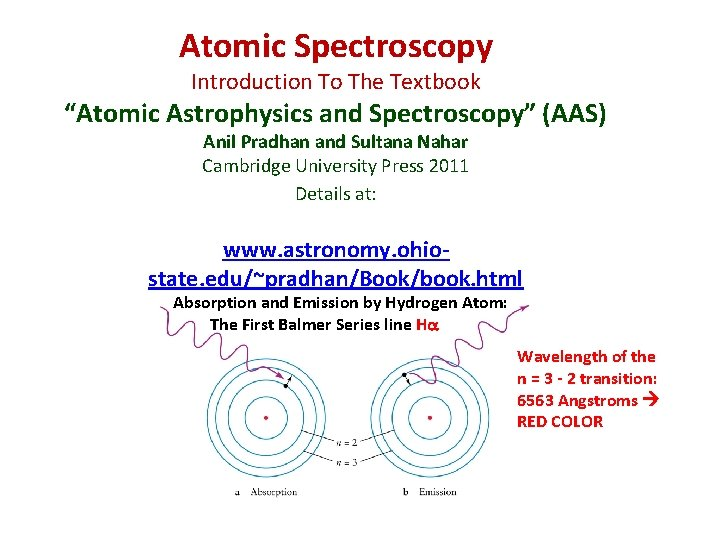Atomic Spectroscopy Introduction To The Textbook “Atomic Astrophysics and Spectroscopy” (AAS) Anil Pradhan and Sultana Nahar Cambridge University Press 2011 Details at: www. astronomy. ohiostate. edu/~pradhan/Book/book. html Absorption and Emission by Hydrogen Atom: The First Balmer Series line Ha Wavelength of the n = 3 - 2 transition: 6563 Angstroms RED COLOR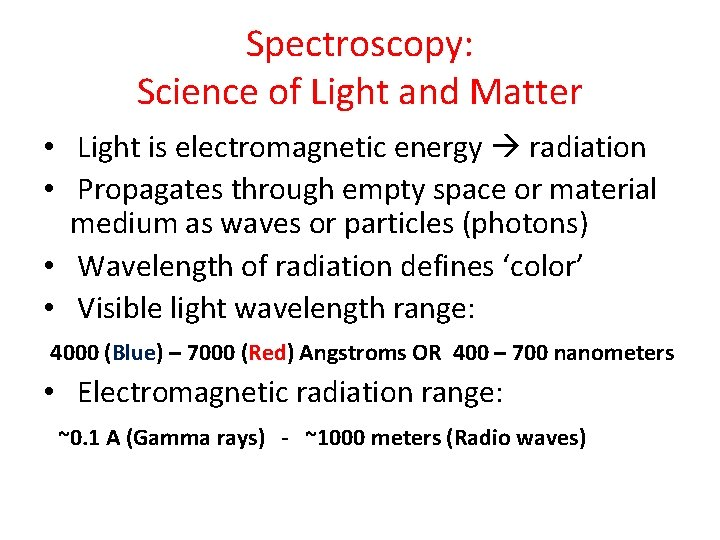Spectroscopy: Science of Light and Matter • Light is electromagnetic energy radiation • Propagates through empty space or material medium as waves or particles (photons) • Wavelength of radiation defines ‘color’ • Visible light wavelength range: 4000 (Blue) – 7000 (Red) Angstroms OR 400 – 700 nanometers • Electromagnetic radiation range: ~0. 1 A (Gamma rays) - ~1000 meters (Radio waves)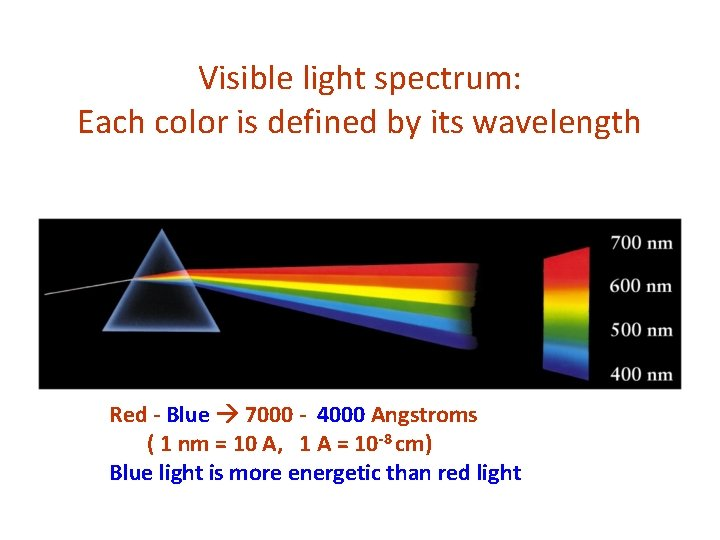Visible light spectrum: Each color is defined by its wavelength Red - Blue 7000 - 4000 Angstroms ( 1 nm = 10 A, 1 A = 10 -8 cm) Blue light is more energetic than red light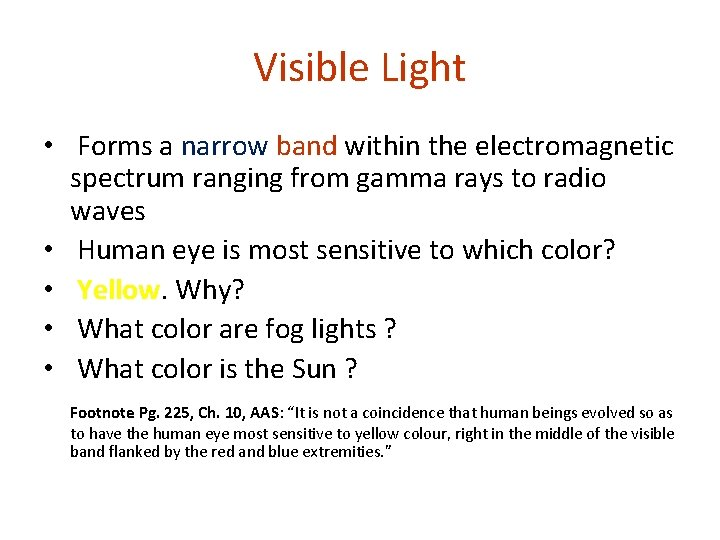Visible Light • Forms a narrow band within the electromagnetic spectrum ranging from gamma rays to radio waves • Human eye is most sensitive to which color? • Yellow. Why? • What color are fog lights ? • What color is the Sun ? Footnote Pg. 225, Ch. 10, AAS: “It is not a coincidence that human beings evolved so as to have the human eye most sensitive to yellow colour, right in the middle of the visible band flanked by the red and blue extremities. ”Light: Electromagnetic Spectrum From Gamma Rays to Radio Waves (cf. Fig. 1. 2, AAS) Gamma rays are the most energetic (highest frequency, shortest wavelength), Radio waves are the least energetic.Light is electromagnetic wave; Does not require a medium to propagate, unlike water or sound Wavelength is the distance between successive crests or troughs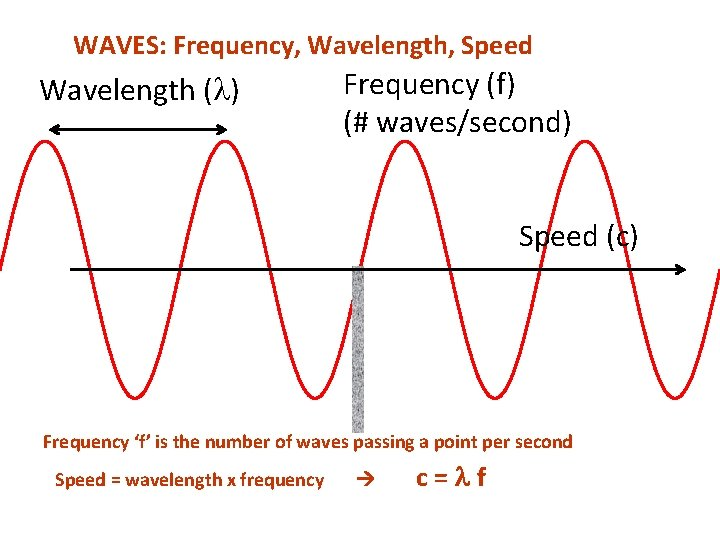WAVES: Frequency, Wavelength, Speed Wavelength ( ) Frequency (f) (# waves/second) Speed (c) Frequency ‘f’ is the number of waves passing a point per second Speed = wavelength x frequency c=lf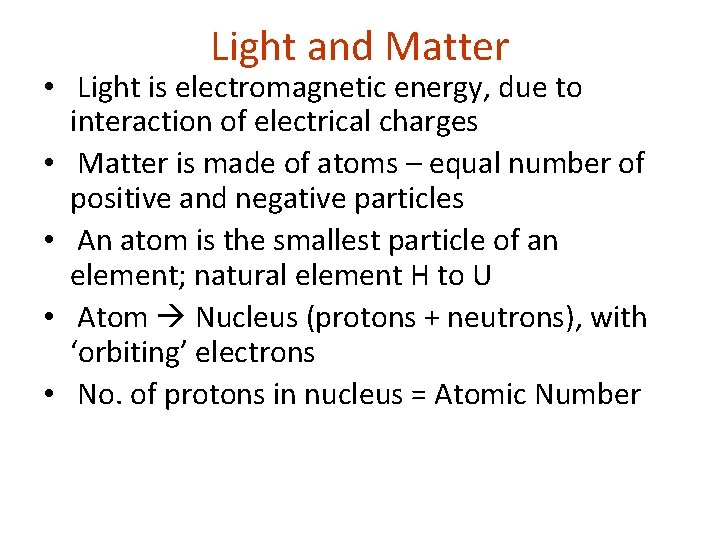Light and Matter • Light is electromagnetic energy, due to interaction of electrical charges • Matter is made of atoms – equal number of positive and negative particles • An atom is the smallest particle of an element; natural element H to U • Atom Nucleus (protons + neutrons), with ‘orbiting’ electrons • No. of protons in nucleus = Atomic Number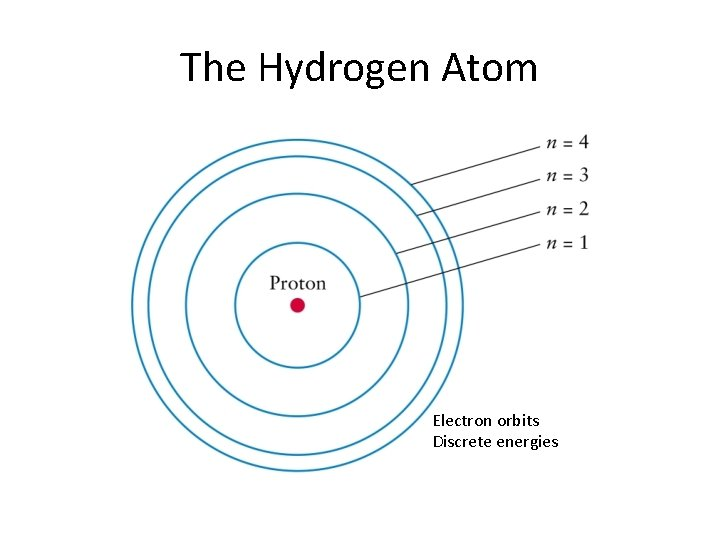The Hydrogen Atom Electron orbits Discrete energiesSeries of spectral lines of HydrogenWavelengths of series of lines from Hydrogen (cf. Fig. 2. 1, AAS)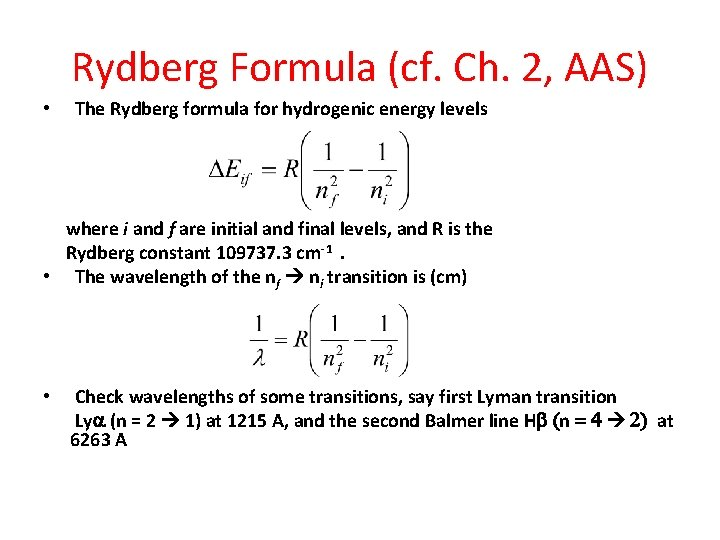Rydberg Formula (cf. Ch. 2, AAS) • The Rydberg formula for hydrogenic energy levels where i and f are initial and final levels, and R is the Rydberg constant 109737. 3 cm-1. • The wavelength of the nf ni transition is (cm) • Check wavelengths of some transitions, say first Lyman transition Lya (n = 2 1) at 1215 A, and the second Balmer line Hb (n = 4 2) at 6263 A